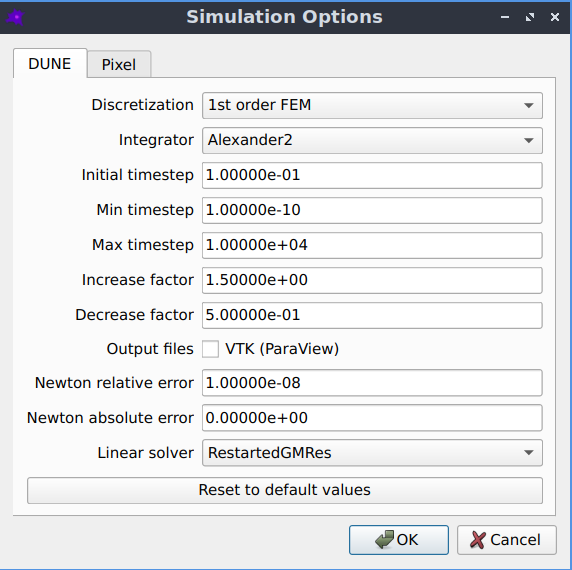# dune-copasi simulator

dune-copasi is the default PDE solver, which solves the PDE on a triangular mesh using finite element discretization methods. The mesh is automatically constructed from the geometry image, as described in Mesh generation.

## Simulation options

The default settings should work well in most cases, but if desired they can be adjusted by going to Advanced->Simulation optionsThe simulation options that can be used to fine tune-the dune-copasi solver.
• Discretization
• currently only 1st order FEM is supported

• other discretizations (such as 2nd order FEM) may be added in the future

• Integrator
• the Runge-Kutta integration scheme used for time integration

• a variety of implicit and explicit schemes of different orders are available

• the default is the 2nd order Alexander scheme, which is a Diagonally Implicit Runge Kutta method

• Initial timestep
• the timestep used at the start of a simulation

• Min timestep
• the minimum allowed timestep

• for a very stiff model it may be necessary to reduce this value

• Max timestep
• the maximum allowed timestep

• reducing this value may increase the accuracy of the solution but simulations will take longer to run

• Increase factor
• after a successful integration step, the timestep is multiplied by this factor

• this must be greater than or equal to 1

• if equal to 1, the timestep is never increased between integration steps

• the larger the value, the more the timestep is increased after successful integration steps

• Decrease factor
• if an integration step is unsuccessful, the timestep is multiplied by this factor and the step is repeated

• this must be less than 1

• the smaller the value, the more the timestep is decreased in the case of an unsuccessful integration step

• Output files
• VTK files of the species concentrations throughout the simulation can be generated

• these files can be viewed using ParaView

• Newton relative error
• the relative error where Newton iteration is considered to have converged

• currently this may need to be altered depending on the units and geometry size (see #315)

• Newton absolute error
• the absolute error where Newton iteration is considered to have converged

• currently this may need to be altered depending on the units and geometry size (see #315)